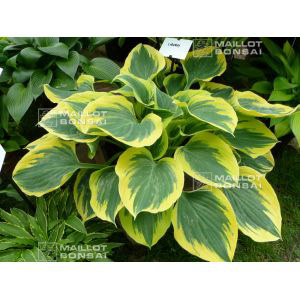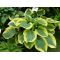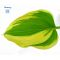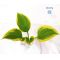##### The Japanese Bonsai specialist
Direct order Contact Help / Services Newsletter# Hosta 'liberty'

Giboshi libertyref. : 6800

8,00

Available quantity : 10Order

###### Description

Big rhizome delivered in a 1.5 litre plastic container. Hosta 'Liberty' has rippled leaves that are blue to dark green in the centre with a margin that changes from yellow to creamy white in summer. Pale lavender flowers bloom from the middle of June to mid-July.

Height: 66 cm x 160 cm wide.
Leaves 25 cm long x 10 cm wide.
Descended from 'Sagae', 2001, seedling.

Named Hosta of the Year 2012 by the American Hosta Growers Association.

#hosta 5.5 #liberty 3.7 #from 3.4 #leaves 2.6 #hostas 2.6 #wide 2.4 #that 2.4 #association 2.1 #container 1.9 #delivered 1.9

Formule
(( ROUND((CHAR_LENGTH(b.article_nom)-CHAR_LENGTH(REPLACE(b.article_nom, 'hosta', '')))/LENGTH('hosta')) + ROUND((CHAR_LENGTH(b.article_description)-CHAR_LENGTH(REPLACE(b.article_description, 'hosta', '')))/LENGTH('hosta')) ) * 5.5) + (( ROUND((CHAR_LENGTH(b.article_nom)-CHAR_LENGTH(REPLACE(b.article_nom, 'liberty', '')))/LENGTH('liberty')) + ROUND((CHAR_LENGTH(b.article_description)-CHAR_LENGTH(REPLACE(b.article_description, 'liberty', '')))/LENGTH('liberty')) ) * 3.7) + (( ROUND((CHAR_LENGTH(b.article_nom)-CHAR_LENGTH(REPLACE(b.article_nom, 'from', '')))/LENGTH('from')) + ROUND((CHAR_LENGTH(b.article_description)-CHAR_LENGTH(REPLACE(b.article_description, 'from', '')))/LENGTH('from')) ) * 3.4) + (( ROUND((CHAR_LENGTH(b.article_nom)-CHAR_LENGTH(REPLACE(b.article_nom, 'leaves', '')))/LENGTH('leaves')) + ROUND((CHAR_LENGTH(b.article_description)-CHAR_LENGTH(REPLACE(b.article_description, 'leaves', '')))/LENGTH('leaves')) ) * 2.6) + (( ROUND((CHAR_LENGTH(b.article_nom)-CHAR_LENGTH(REPLACE(b.article_nom, 'wide', '')))/LENGTH('wide')) + ROUND((CHAR_LENGTH(b.article_description)-CHAR_LENGTH(REPLACE(b.article_description, 'wide', '')))/LENGTH('wide')) ) * 2.4) + (( ROUND((CHAR_LENGTH(b.article_nom)-CHAR_LENGTH(REPLACE(b.article_nom, 'that', '')))/LENGTH('that')) + ROUND((CHAR_LENGTH(b.article_description)-CHAR_LENGTH(REPLACE(b.article_description, 'that', '')))/LENGTH('that')) ) * 2.4) + (( ROUND((CHAR_LENGTH(b.article_nom)-CHAR_LENGTH(REPLACE(b.article_nom, 'association', '')))/LENGTH('association')) + ROUND((CHAR_LENGTH(b.article_description)-CHAR_LENGTH(REPLACE(b.article_description, 'association', '')))/LENGTH('association')) ) * 2.1) + (( ROUND((CHAR_LENGTH(b.article_nom)-CHAR_LENGTH(REPLACE(b.article_nom, 'container', '')))/LENGTH('container')) + ROUND((CHAR_LENGTH(b.article_description)-CHAR_LENGTH(REPLACE(b.article_description, 'container', '')))/LENGTH('container')) ) * 1.9) + (( ROUND((CHAR_LENGTH(b.article_nom)-CHAR_LENGTH(REPLACE(b.article_nom, 'delivered', '')))/LENGTH('delivered')) + ROUND((CHAR_LENGTH(b.article_description)-CHAR_LENGTH(REPLACE(b.article_description, 'delivered', '')))/LENGTH('delivered')) ) * 1.9) + (( ROUND((CHAR_LENGTH(b.article_nom)-CHAR_LENGTH(REPLACE(b.article_nom, 'descended', '')))/LENGTH('descended')) + ROUND((CHAR_LENGTH(b.article_description)-CHAR_LENGTH(REPLACE(b.article_description, 'descended', '')))/LENGTH('descended')) ) * 1.9)

## Secure payment## Delivery

Our logistic partners :04 74 55 23 48
Pépinière MAILLOT-BONSAÏ
Le Bois Frazy
01990 RELEVANT - FRANCE
on appointment